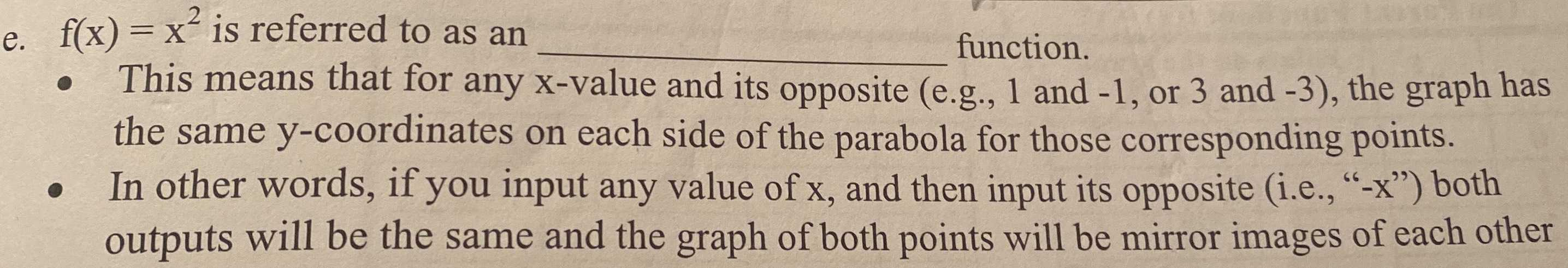### Still have math questions?

Algebra
Question$$f ( x ) = x ^ { 2 }$$ is referred to as an function.

This means that for any $$x$$ -value and its opposite (e.g., $$1$$ and $$- 1$$ , or $$3$$ and $$- 3$$ ), the graph has

the same $$y$$ -coordinates on each side of the parabola for those corresponding points.

In other words, if you input any value of $$x ,$$ and then input its opposite (i.e., "-x") both outputs will be the same and the graph of both points will be mirror images of each other